# Selina Solutions Concise Mathematics Class 6 Chapter 21 Framing Algebraic Expressions (Including Evaluation)

Selina Solutions Concise Mathematics Class 6 Chapter 21 Framing Algebraic Expressions (Including Evaluation) are well structured by subject matter experts, with the intention to help students ace the exam. Students, who aspire to enhance problem-solving skills, can make use of solutions on a regular basis. Regular practice of these solutions helps students to overcome their confusion about the concepts and hence understand in-depth. For securing more marks in the annual examination of Class 6, students can use Selina Solutions Concise Mathematics Class 6 Chapter 21 Framing Algebraic Expressions (Including Evaluation) PDF, from the links which are available for free download.

Chapter 21 Framing Algebraic Expressions (Including Evaluation) explains each and every question based on framing algebraic expression along with the evaluation in detail. Practicing the solved examples from the chapter using the provided solutions helps students in solving the complex problems effortlessly, within a shorter duration.

## Selina Solutions Concise Mathematics Class 6 Chapter 21 Framing Algebraic Expressions (Including Evaluation) Download PDF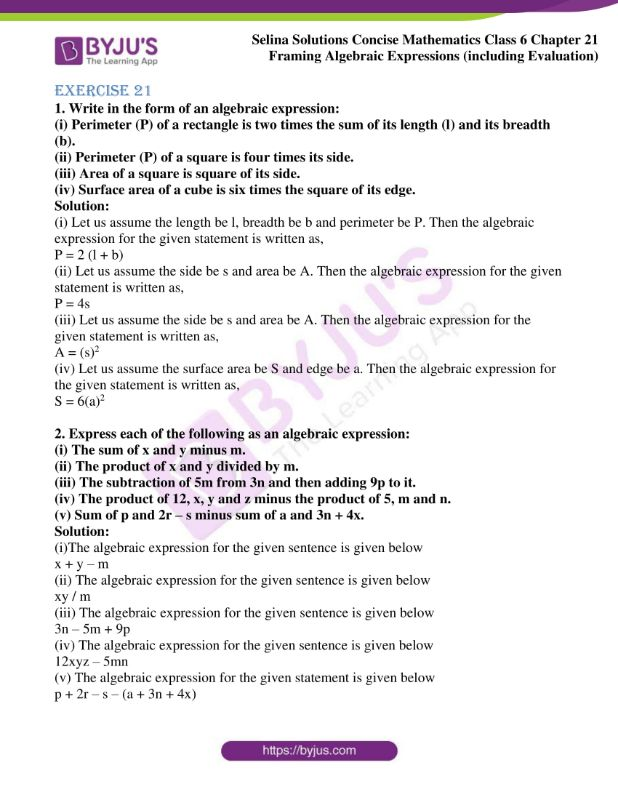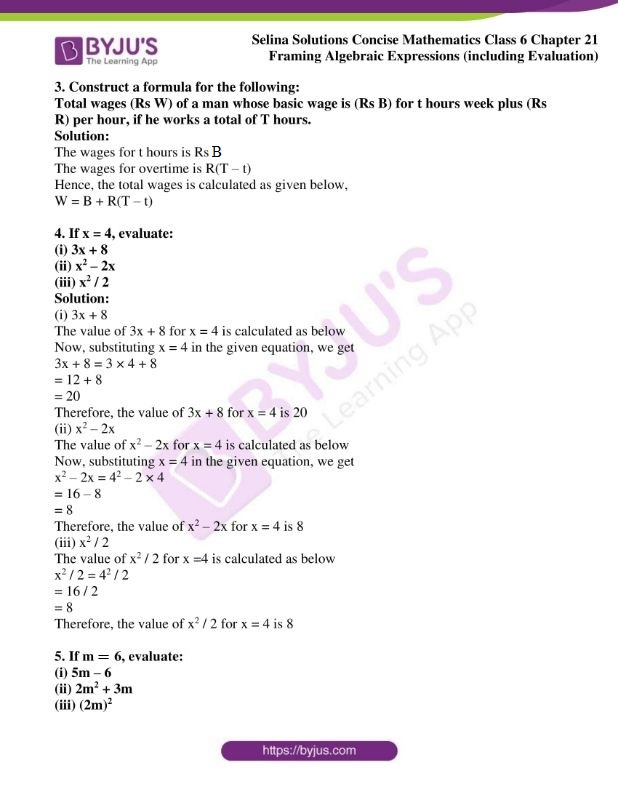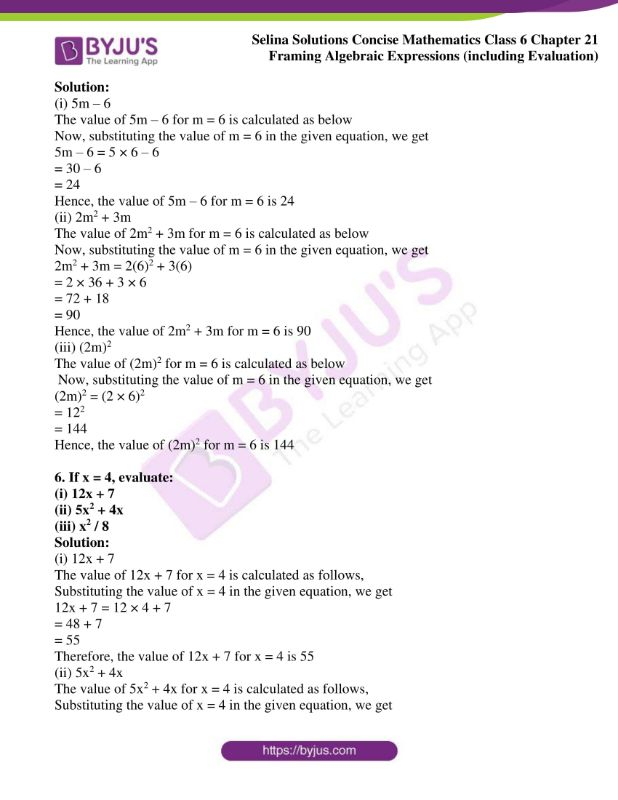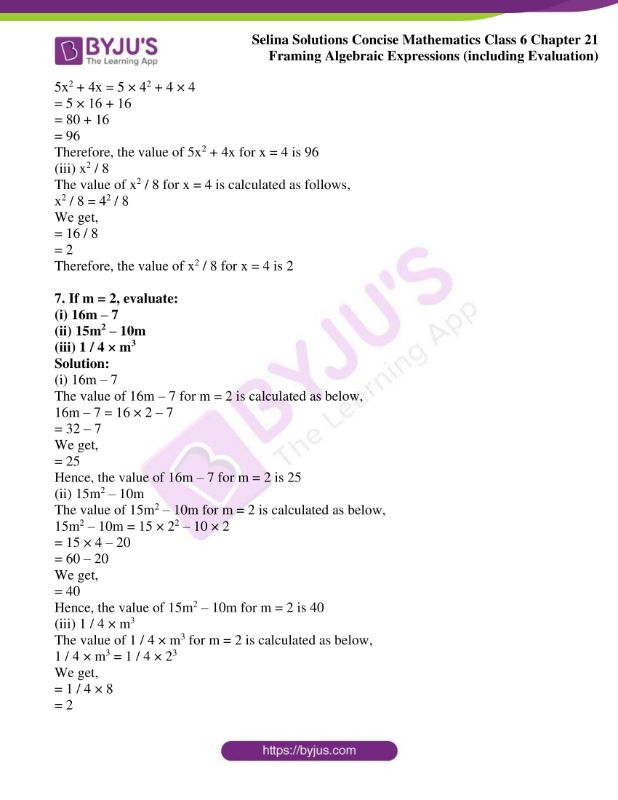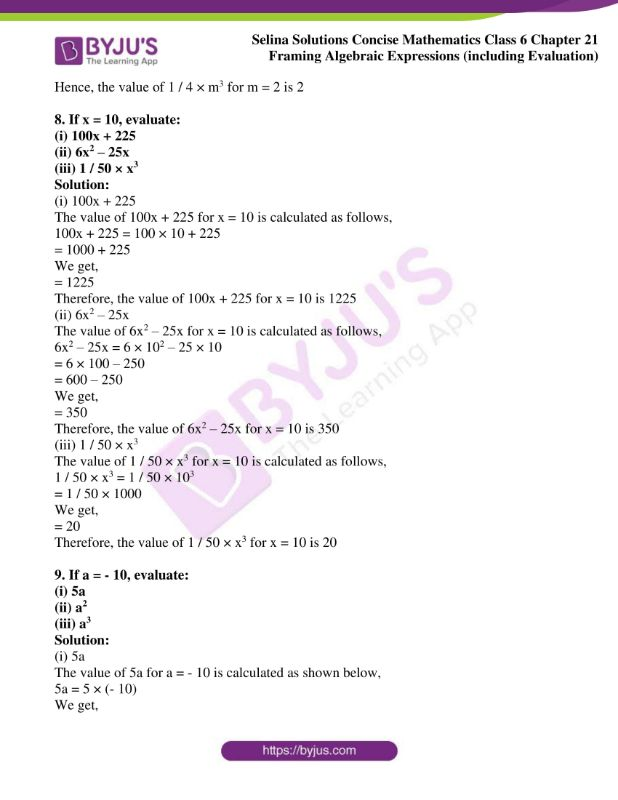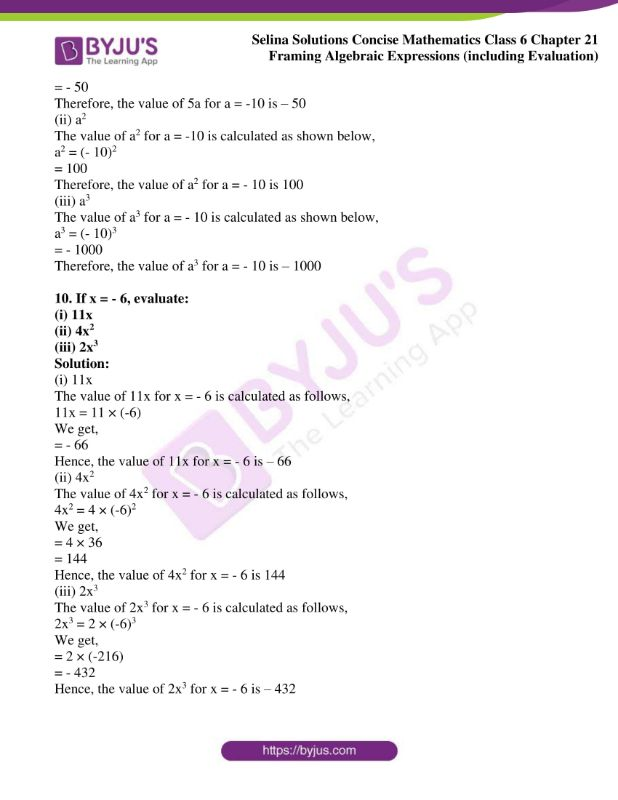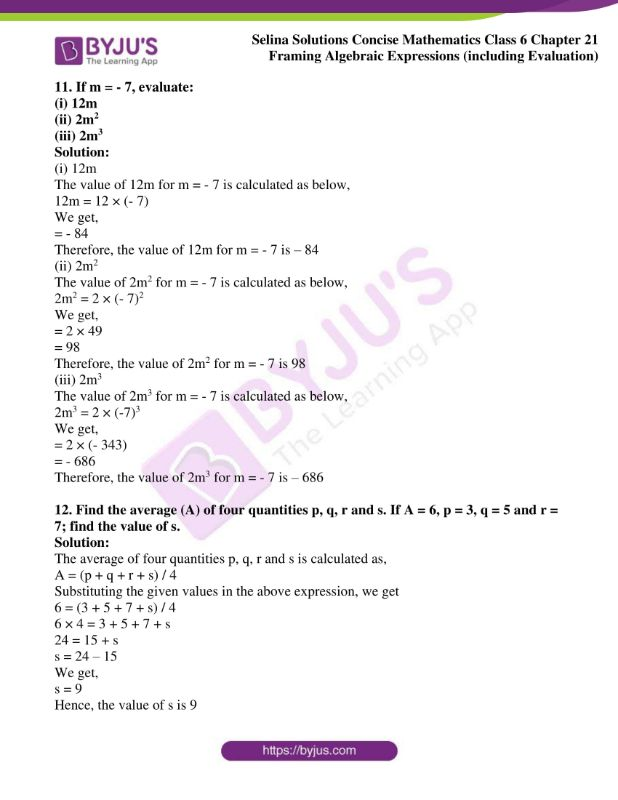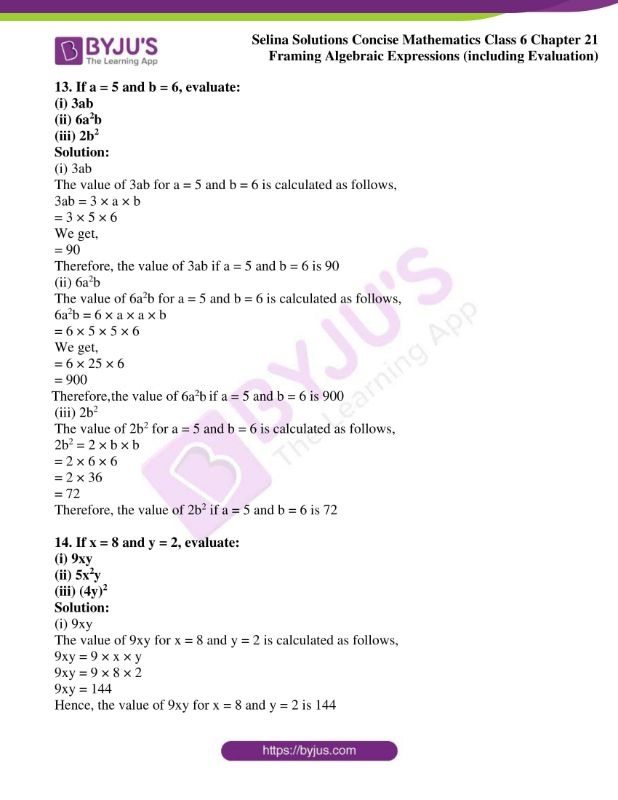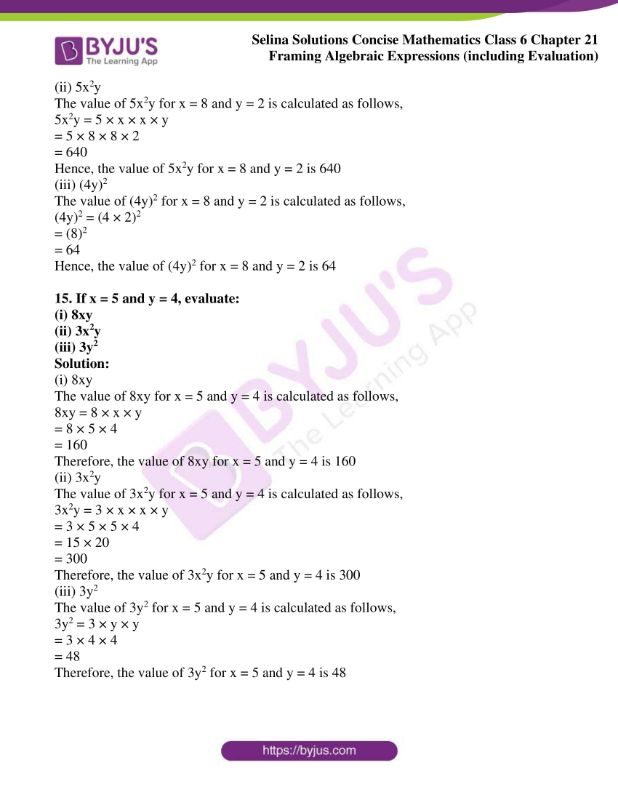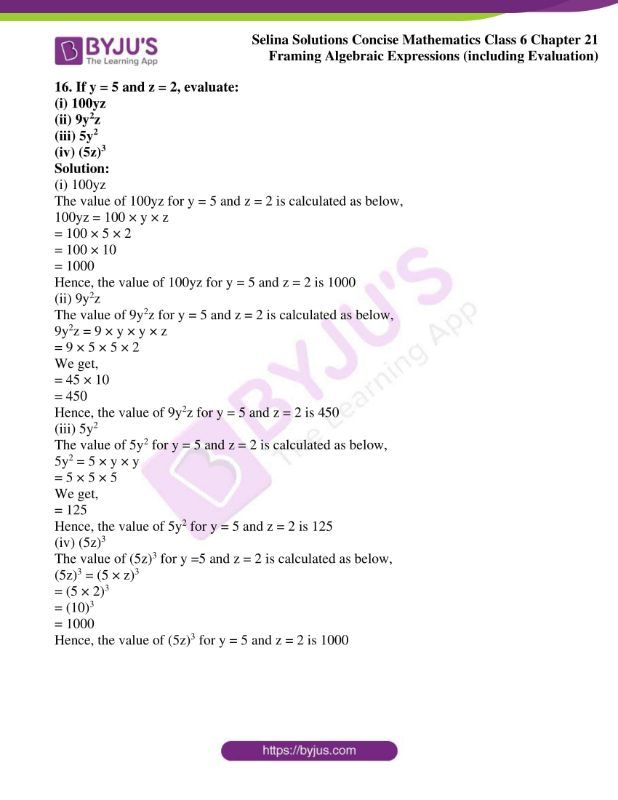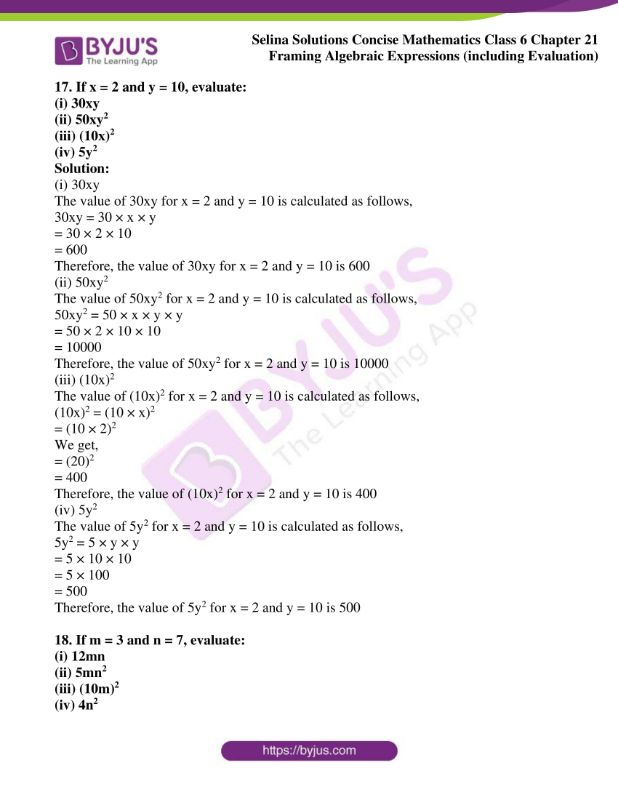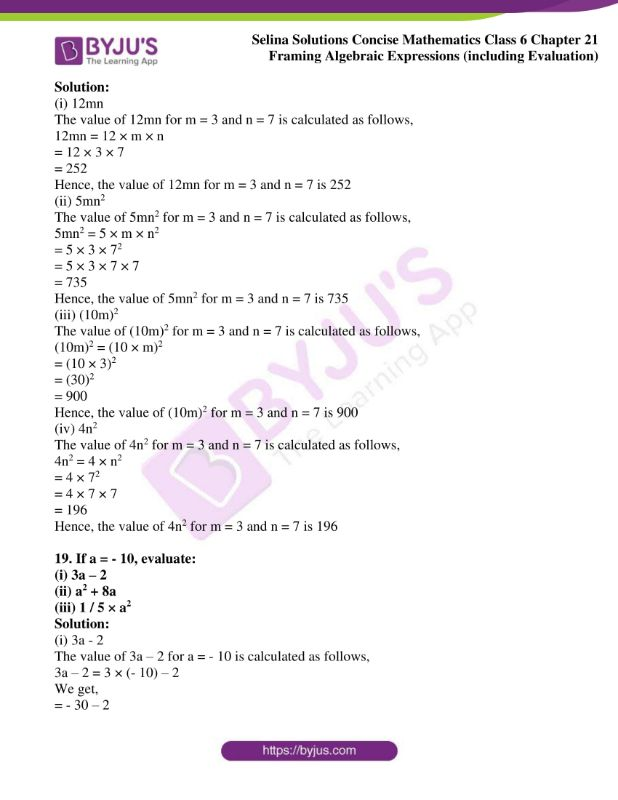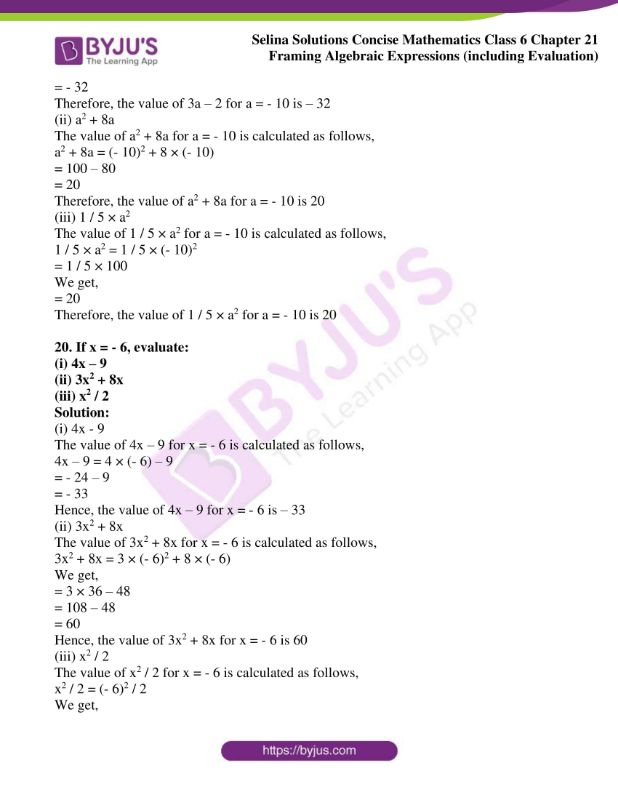### Access Selina Solutions Concise Mathematics Class 6 Chapter 21 Framing Algebraic Expressions (Including Evaluation)

Exercise 21

1. Write in the form of an algebraic expression:

(i) Perimeter (P) of a rectangle is two times the sum of its length (l) and its breadth (b).

(ii) Perimeter (P) of a square is four times its side.

(iii) Area of a square is square of its side.

(iv) Surface area of a cube is six times the square of its edge.

Solution:

(i) Let us assume the length be l, breadth be b and perimeter be P. Then the algebraic expression for the given statement is written as,

P = 2 (l + b)

(ii) Let us assume the side be s and area be A. Then the algebraic expression for the given statement is written as,

P = 4s

(iii) Let us assume the side be s and area be A. Then the algebraic expression for the given statement is written as,

A = (s)2

(iv) Let us assume the surface area be S and edge be a. Then the algebraic expression for the given statement is written as,

S = 6(a)2

2. Express each of the following as an algebraic expression:

(i) The sum of x and y minus m.

(ii) The product of x and y divided by m.

(iii) The subtraction of 5m from 3n and then adding 9p to it.

(iv) The product of 12, x, y and z minus the product of 5, m and n.

(v) Sum of p and 2r – s minus sum of a and 3n + 4x.

Solution:

(i)The algebraic expression for the given sentence is given below

x + y – m

(ii) The algebraic expression for the given sentence is given below

xy / m

(iii) The algebraic expression for the given sentence is given below

3n – 5m + 9p

(iv) The algebraic expression for the given sentence is given below

12xyz – 5mn

(v) The algebraic expression for the given statement is given below

p + 2r – s – (a + 3n + 4x)

3. Construct a formula for the following:

Total wages (Rs W) of a man whose basic wage is (Rs B) for t hours week plus (Rs R) per hour, if he works a total of T hours.

Solution:

The wages for t hours is Rs B.

The wages for overtime is R(T – t)

Hence, the total wages is calculated as given below,

W = B + R(T – t)

4. If x = 4, evaluate:

(i) 3x + 8

(ii) x2 – 2x

(iii) x2 / 2

Solution:

(i) 3x + 8

The value of 3x + 8 for x = 4 is calculated as below

Now, substituting x = 4 in the given equation, we get

3x + 8 = 3 × 4 + 8

= 12 + 8

= 20

Therefore, the value of 3x + 8 for x = 4 is 20

(ii) x2 – 2x

The value of x2 – 2x for x = 4 is calculated as below

Now, substituting x = 4 in the given equation, we get

x2 – 2x = 42 – 2 × 4

= 16 – 8

= 8

Therefore, the value of x2 – 2x for x = 4 is 8

(iii) x2 / 2

The value of x2 / 2 for x =4 is calculated as below

x2 / 2 = 42 / 2

= 16 / 2

= 8

Therefore, the value of x2 / 2 for x = 4 is 8

5. If m = 6, evaluate:

(i) 5m – 6

(ii) 2m2 + 3m

(iii) (2m)2

Solution:

(i) 5m – 6

The value of 5m – 6 for m = 6 is calculated as below

Now, substituting the value of m = 6 in the given equation, we get

5m – 6 = 5 × 6 – 6

= 30 – 6

= 24

Hence, the value of 5m – 6 for m = 6 is 24

(ii) 2m2 + 3m

The value of 2m2 + 3m for m = 6 is calculated as below

Now, substituting the value of m = 6 in the given equation, we get

2m2 + 3m = 2(6)2 + 3(6)

= 2 × 36 + 3 × 6

= 72 + 18

= 90

Hence, the value of 2m2 + 3m for m = 6 is 90

(iii) (2m)2

The value of (2m)2 for m = 6 is calculated as below

Now, substituting the value of m = 6 in the given equation, we get

(2m)2 = (2 × 6)2

= 122

= 144

Hence, the value of (2m)2 for m = 6 is 144

6. If x = 4, evaluate:

(i) 12x + 7

(ii) 5x2 + 4x

(iii) x2 / 8

Solution:

(i) 12x + 7

The value of 12x + 7 for x = 4 is calculated as follows,

Substituting the value of x = 4 in the given equation, we get

12x + 7 = 12 × 4 + 7

= 48 + 7

= 55

Therefore, the value of 12x + 7 for x = 4 is 55

(ii) 5x2 + 4x

The value of 5x2 + 4x for x = 4 is calculated as follows,

Substituting the value of x = 4 in the given equation, we get

5x2 + 4x = 5 × 42 + 4 × 4

= 5 × 16 + 16

= 80 + 16

= 96

Therefore, the value of 5x2 + 4x for x = 4 is 96

(iii) x2 / 8

The value of x2 / 8 for x = 4 is calculated as follows,

x2 / 8 = 42 / 8

We get,

= 16 / 8

= 2

Therefore, the value of x2 / 8 for x = 4 is 2

7. If m = 2, evaluate:

(i) 16m – 7

(ii) 15m2 – 10m

(iii) 1 / 4 × m3

Solution:

(i) 16m – 7

The value of 16m – 7 for m = 2 is calculated as below,

16m – 7 = 16 × 2 – 7

= 32 – 7

We get,

= 25

Hence, the value of 16m – 7 for m = 2 is 25

(ii) 15m2 – 10m

The value of 15m2 – 10m for m = 2 is calculated as below,

15m2 – 10m = 15 × 22 – 10 × 2

= 15 × 4 – 20

= 60 – 20

We get,

= 40

Hence, the value of 15m2 – 10m for m = 2 is 40

(iii) 1 / 4 × m3

The value of 1 / 4 × m3 for m = 2 is calculated as below,

1 / 4 × m3 = 1 / 4 × 23

We get,

= 1 / 4 × 8

= 2

Hence, the value of 1 / 4 × m3 for m = 2 is 2

8. If x = 10, evaluate:

(i) 100x + 225

(ii) 6x2 – 25x

(iii) 1 / 50 × x3

Solution:

(i) 100x + 225

The value of 100x + 225 for x = 10 is calculated as follows,

100x + 225 = 100 × 10 + 225

= 1000 + 225

We get,

= 1225

Therefore, the value of 100x + 225 for x = 10 is 1225

(ii) 6x2 – 25x

The value of 6x2 – 25x for x = 10 is calculated as follows,

6x2 – 25x = 6 × 102 – 25 × 10

= 6 × 100 – 250

= 600 – 250

We get,

= 350

Therefore, the value of 6x2 – 25x for x = 10 is 350

(iii) 1 / 50 × x3

The value of 1 / 50 × x3 for x = 10 is calculated as follows,

1 / 50 × x3 = 1 / 50 × 103

= 1 / 50 × 1000

We get,

= 20

Therefore, the value of 1 / 50 × x3 for x = 10 is 20

9. If a = – 10, evaluate:

(i) 5a

(ii) a2

(iii) a3

Solution:

(i) 5a

The value of 5a for a = – 10 is calculated as shown below,

5a = 5 × (- 10)

We get,

= – 50

Therefore, the value of 5a for a = -10 is – 50

(ii) a2

The value of a2 for a = -10 is calculated as shown below,

a2 = (- 10)2

= 100

Therefore, the value of a2 for a = – 10 is 100

(iii) a3

The value of a3 for a = – 10 is calculated as shown below,

a3 = (- 10)3

= – 1000

Therefore, the value of a3 for a = – 10 is – 1000

10. If x = – 6, evaluate:

(i) 11x

(ii) 4x2

(iii) 2x3

Solution:

(i) 11x

The value of 11x for x = – 6 is calculated as follows,

11x = 11 × (-6)

We get,

= – 66

Hence, the value of 11x for x = – 6 is – 66

(ii) 4x2

The value of 4x2 for x = – 6 is calculated as follows,

4x2 = 4 × (-6)2

We get,

= 4 × 36

= 144

Hence, the value of 4x2 for x = – 6 is 144

(iii) 2x3

The value of 2x3 for x = – 6 is calculated as follows,

2x3 = 2 × (-6)3

We get,

= 2 × (-216)

= – 432

Hence, the value of 2x3 for x = – 6 is – 432

11. If m = – 7, evaluate:

(i) 12m

(ii) 2m2

(iii) 2m3

Solution:

(i) 12m

The value of 12m for m = – 7 is calculated as below,

12m = 12 × (- 7)

We get,

= – 84

Therefore, the value of 12m for m = – 7 is – 84

(ii) 2m2

The value of 2m2 for m = – 7 is calculated as below,

2m2 = 2 × (- 7)2

We get,

= 2 × 49

= 98

Therefore, the value of 2m2 for m = – 7 is 98

(iii) 2m3

The value of 2m3 for m = – 7 is calculated as below,

2m3 = 2 × (-7)3

We get,

= 2 × (- 343)

= – 686

Therefore, the value of 2m3 for m = – 7 is – 686

12. Find the average (A) of four quantities p, q, r and s. If A = 6, p = 3, q = 5 and r = 7; find the value of s.

Solution:

The average of four quantities p, q, r and s is calculated as,

A = (p + q + r + s) / 4

Substituting the given values in the above expression, we get

6 = (3 + 5 + 7 + s) / 4

6 × 4 = 3 + 5 + 7 + s

24 = 15 + s

s = 24 – 15

We get,

s = 9

Hence, the value of s is 9

13. If a = 5 and b = 6, evaluate:

(i) 3ab

(ii) 6a2b

(iii) 2b2

Solution:

(i) 3ab

The value of 3ab for a = 5 and b = 6 is calculated as follows,

3ab = 3 × a × b

= 3 × 5 × 6

We get,

= 90

Therefore, the value of 3ab if a = 5 and b = 6 is 90

(ii) 6a2b

The value of 6a2b for a = 5 and b = 6 is calculated as follows,

6a2b = 6 × a × a × b

= 6 × 5 × 5 × 6

We get,

= 6 × 25 × 6

= 900

Therefore, the value of 6a2b if a = 5 and b = 6 is 900

(iii) 2b2

The value of 2b2 for a = 5 and b = 6 is calculated as follows,

2b2 = 2 × b × b

= 2 × 6 × 6

= 2 × 36

= 72

Therefore, the value of 2b2 if a = 5 and b = 6 is 72

14. If x = 8 and y = 2, evaluate:

(i) 9xy

(ii) 5x2y

(iii) (4y)2

Solution:

(i) 9xy

The value of 9xy for x = 8 and y = 2 is calculated as follows,

9xy = 9 × x × y

9xy = 9 × 8 × 2

9xy = 144

Hence, the value of 9xy for x = 8 and y = 2 is 144

(ii) 5x2y

The value of 5x2y for x = 8 and y = 2 is calculated as follows,

5x2y = 5 × x × x × y

= 5 × 8 × 8 × 2

= 640

Hence, the value of 5x2y for x = 8 and y = 2 is 640

(iii) (4y)2

The value of (4y)2 for x = 8 and y = 2 is calculated as follows,

(4y)2 = (4 × 2)2

= (8)2

= 64

Hence, the value of (4y)2 for x = 8 and y = 2 is 64

15. If x = 5 and y = 4, evaluate:

(i) 8xy

(ii) 3x2y

(iii) 3y2

Solution:

(i) 8xy

The value of 8xy for x = 5 and y = 4 is calculated as follows,

8xy = 8 × x × y

= 8 × 5 × 4

= 160

Therefore, the value of 8xy for x = 5 and y = 4 is 160

(ii) 3x2y

The value of 3x2y for x = 5 and y = 4 is calculated as follows,

3x2y = 3 × x × x × y

= 3 × 5 × 5 × 4

= 15 × 20

= 300

Therefore, the value of 3x2y for x = 5 and y = 4 is 300

(iii) 3y2

The value of 3y2 for x = 5 and y = 4 is calculated as follows,

3y2 = 3 × y × y

= 3 × 4 × 4

= 48

Therefore, the value of 3y2 for x = 5 and y = 4 is 48

16. If y = 5 and z = 2, evaluate:

(i) 100yz

(ii) 9y2z

(iii) 5y2

(iv) (5z)3

Solution:

(i) 100yz

The value of 100yz for y = 5 and z = 2 is calculated as below,

100yz = 100 × y × z

= 100 × 5 × 2

= 100 × 10

= 1000

Hence, the value of 100yz for y = 5 and z = 2 is 1000

(ii) 9y2z

The value of 9y2z for y = 5 and z = 2 is calculated as below,

9y2z = 9 × y × y × z

= 9 × 5 × 5 × 2

We get,

= 45 × 10

= 450

Hence, the value of 9y2z for y = 5 and z = 2 is 450

(iii) 5y2

The value of 5y2 for y = 5 and z = 2 is calculated as below,

5y2 = 5 × y × y

= 5 × 5 × 5

We get,

= 125

Hence, the value of 5y2 for y = 5 and z = 2 is 125

(iv) (5z)3

The value of (5z)3 for y =5 and z = 2 is calculated as below,

(5z)3 = (5 × z)3

= (5 × 2)3

= (10)3

= 1000

Hence, the value of (5z)3 for y = 5 and z = 2 is 1000

17. If x = 2 and y = 10, evaluate:

(i) 30xy

(ii) 50xy2

(iii) (10x)2

(iv) 5y2

Solution:

(i) 30xy

The value of 30xy for x = 2 and y = 10 is calculated as follows,

30xy = 30 × x × y

= 30 × 2 × 10

= 600

Therefore, the value of 30xy for x = 2 and y = 10 is 600

(ii) 50xy2

The value of 50xy2 for x = 2 and y = 10 is calculated as follows,

50xy2 = 50 × x × y × y

= 50 × 2 × 10 × 10

= 10000

Therefore, the value of 50xy2 for x = 2 and y = 10 is 10000

(iii) (10x)2

The value of (10x)2 for x = 2 and y = 10 is calculated as follows,

(10x)2 = (10 × x)2

= (10 × 2)2

We get,

= (20)2

= 400

Therefore, the value of (10x)2 for x = 2 and y = 10 is 400

(iv) 5y2

The value of 5y2 for x = 2 and y = 10 is calculated as follows,

5y2 = 5 × y × y

= 5 × 10 × 10

= 5 × 100

= 500

Therefore, the value of 5y2 for x = 2 and y = 10 is 500

18. If m = 3 and n = 7, evaluate:

(i) 12mn

(ii) 5mn2

(iii) (10m)2

(iv) 4n2

Solution:

(i) 12mn

The value of 12mn for m = 3 and n = 7 is calculated as follows,

12mn = 12 × m × n

= 12 × 3 × 7

= 252

Hence, the value of 12mn for m = 3 and n = 7 is 252

(ii) 5mn2

The value of 5mn2 for m = 3 and n = 7 is calculated as follows,

5mn2 = 5 × m × n2

= 5 × 3 × 72

= 5 × 3 × 7 × 7

= 735

Hence, the value of 5mn2 for m = 3 and n = 7 is 735

(iii) (10m)2

The value of (10m)2 for m = 3 and n = 7 is calculated as follows,

(10m)2 = (10 × m)2

= (10 × 3)2

= (30)2

= 900

Hence, the value of (10m)2 for m = 3 and n = 7 is 900

(iv) 4n2

The value of 4n2 for m = 3 and n = 7 is calculated as follows,

4n2 = 4 × n2

= 4 × 72

= 4 × 7 × 7

= 196

Hence, the value of 4n2 for m = 3 and n = 7 is 196

19. If a = – 10, evaluate:

(i) 3a – 2

(ii) a2 + 8a

(iii) 1 / 5 × a2

Solution:

(i) 3a – 2

The value of 3a – 2 for a = – 10 is calculated as follows,

3a – 2 = 3 × (- 10) – 2

We get,

= – 30 – 2

= – 32

Therefore, the value of 3a – 2 for a = – 10 is – 32

(ii) a2 + 8a

The value of a2 + 8a for a = – 10 is calculated as follows,

a2 + 8a = (- 10)2 + 8 × (- 10)

= 100 – 80

= 20

Therefore, the value of a2 + 8a for a = – 10 is 20

(iii) 1 / 5 × a2

The value of 1 / 5 × a2 for a = – 10 is calculated as follows,

1 / 5 × a2 = 1 / 5 × (- 10)2

= 1 / 5 × 100

We get,

= 20

Therefore, the value of 1 / 5 × a2 for a = – 10 is 20

20. If x = – 6, evaluate:

(i) 4x – 9

(ii) 3x2 + 8x

(iii) x2 / 2

Solution:

(i) 4x – 9

The value of 4x – 9 for x = – 6 is calculated as follows,

4x – 9 = 4 × (- 6) – 9

= – 24 – 9

= – 33

Hence, the value of 4x – 9 for x = – 6 is – 33

(ii) 3x2 + 8x

The value of 3x2 + 8x for x = – 6 is calculated as follows,

3x2 + 8x = 3 × (- 6)2 + 8 × (- 6)

We get,

= 3 × 36 – 48

= 108 – 48

= 60

Hence, the value of 3x2 + 8x for x = – 6 is 60

(iii) x2 / 2

The value of x2 / 2 for x = – 6 is calculated as follows,

x2 / 2 = (- 6)2 / 2

We get,

= 36 / 2

= 18

Hence, the value of x2 / 2 for x = – 6 is 18